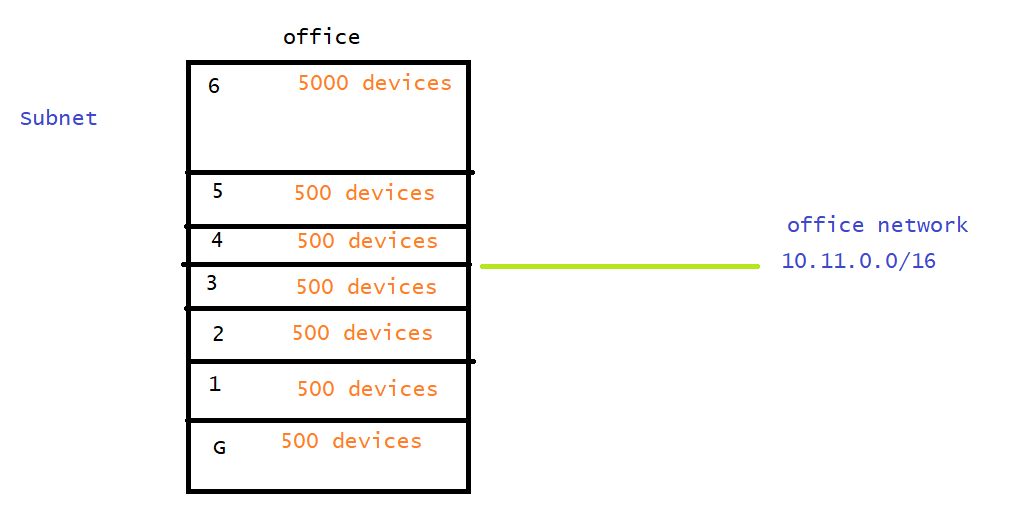### Networking contd

• Now lets create a network which can accomodate 2000 devices Refer Here for table of values
``````hosts size = 2000
number of bits for host

2^n ~= 2000
n = 11

: x.x.x.x/21
: 10.0.0.0/21 or
: 172.16.0.0/21
: 192.168.0.0/21

255.255.248.0
``````
• Generally we choose ip address which are reserved for private networking. private cidrs are
• 10.0.0.0/8
• 172.16.0.0/12
• 192.168.0.0/16
• Lets try to create a network which can accomodate 100000 devices
``````host size = 100000
2^n ~= 100000
n = 17

: 10.0.0.0/15
:172.16.0.0/15

255.254.0.0
``````
• Generally we will not create one huge network, we will break huge network into multiple smaller sub networks, and this is called as subnet. Subnet should have network id which is equal or greater than main network
``````network: 10.10.0.0/16  => 2^16 => network id : 10.10
correct subnet: 10.10.0.0/24 => 2^8 => network id => 10.10.0
correct subnet: 10.10.0.0/16 => 2^16 network id: 10.10
wrong subnet: 10.10.0.0/15  => 2^17
``````
• Lets try to create 3 subnets of size to connect 200 devices in a network with range 192.168.0.0/16
``````network : 192.168.0.0/16

host size ~= 200
2^n ~=200
n = 8

subnet1:

subnet2:
192.168.1.0/24

subnet3:
192.168.2.0/24

``````
• Lets try to create 3 subnets subnet1 with 200 devices subnet2 and subnet 3with 500 devices from a network of 172.16.0.0/16
``````network: 172.16.0.0/16

subnet1 :
host size: 200
2^n ~= 200
n = 8
subnet: 172.16.0.0/24

subnet2:
host size: 500
2^n ~= 500
n = 9
172.16.2.0/23

subnet3:
host size: 500
2^n ~= 500
n = 9
172.16.4.0/23
``````
• Lets try to create 4 subnets of size 1000 each in the network 192.168.0.0/20
``````network: 192.168.0.0/20
subnet with 1000
2^n ~= 1000
n = 10

192.168.0000    00    00. 00000000

subnet1 = 192.168.0.0/22

subnet2 = 192.168.4.0/22

subnet3 = 192.168.8.0/22

subnet4 = 192.168.12.0/22
``````
• Exercise: Create a subnets for each floor as mentioned in the below image### Router

• Router is a networking component which can forward packets from one network to other``````tracert google.com